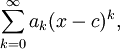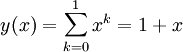# The Power Series

Previous: A Motivating Problem for Power Series

Next: Power Series Example

Indeed, a there is a more general representation for the series we introduced on the previous page:The more general representation is given by the following definition.

Definition: Power Series
An infinite series of the formwhere c is a constant, is a power series about c. The constants, ak are referred to as the coefficients of the series.

### What Is A Power Series?

It may help to consider a simple example with partial sums. Let's take the case where ak = 1, and c = 0, which gives usIf we let N = 1, then we have a polynomial of order 1If we take N = 2, then we have a polynomial of order 2Similarly, with N = 3, then we would obtain a polynomial of order 3In the limit as N goes to infinity, we obtain a power series.

The figure below provides a graphical explanation of what a power series is: an infinite polynomial.This diagram helps to demonstrate the idea of a what a power series is. We consider the simple case when ak = 1, and c = 0. Using partial sums, the left corresponds to N = 1, and the right to N = 2. As we let N go to infinity, we obtain a power series.

Previous: A Motivating Problem for Power Series

Next: Power Series Example